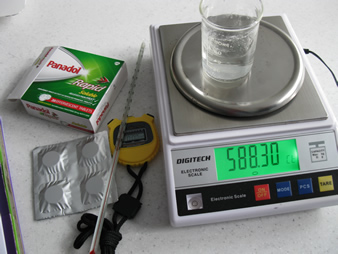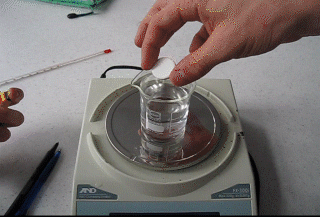How does temperature influence the rate of a reaction?
How long will it take to dissolve an effervescent Panadol tablet in water at 100oC?

When panadol tablets dissolve a chemical reaction takes place that releases carbon dioxide.
Equipment needed for this investigation is shown on the right. Access to hot water is also required via a hot water urn.
- ice
- thermometer
- 200mL beaker
- stop watch
- electronic balance

In this investigation students are aksed to find the time taken for a Panadol tablet to dissolve in water at a temperature of 100oC. The water supplied is not at 100oC hence students collect data and produce a model for the dissolving of Panadol in water of a given temperature. This model is then used to answer the question.The rate of the reaction can be measured by measuring the rate at which mass is lost.

Dissolve a number of Panadol tablets at a range of temperatures and record the time it takes for the tablet to be completely dissolved. Record your results in the table below.
It is up to the student to determine the range of temperatures to be tested.

The electronic scales are not necessary for this part of the investigation but necessary for an extension to measure the imapct of surface area to rate of reaction.Time (Sec) Temperature (oC)
Graph the results on a set of axes drawn on the graph paper on the left.
Things to consider when drawing a graph.
- Dependent variable = y-axis
- Independnet variable = x-axis
- Evenly spaced units on each axis
- Units labelled on each axis.
- Accurately plotted data points.
- Line of best fit ignoring outliers.1) Outline and explain a testable hypothesis based on correct scientific reasoning.
2) State the dependent and independent variables. Describe how the variables were measured or manipulated.
3) Using your graph predict the time taken for a tablet to be completely dissolved at 100oC and 60oC
4) How accurate are the predicitons in question 2 above?
5) What are the controlled variables?
6) Suggest how the controlled variables will be manipulated and why it is necessary to control them.
7) Design a safe, logical sequence of steps that forms the method for this investigation.

Solution

Application scenario